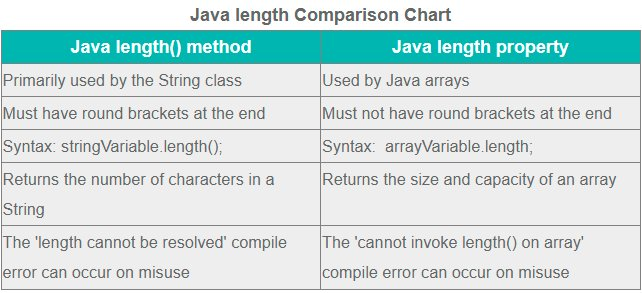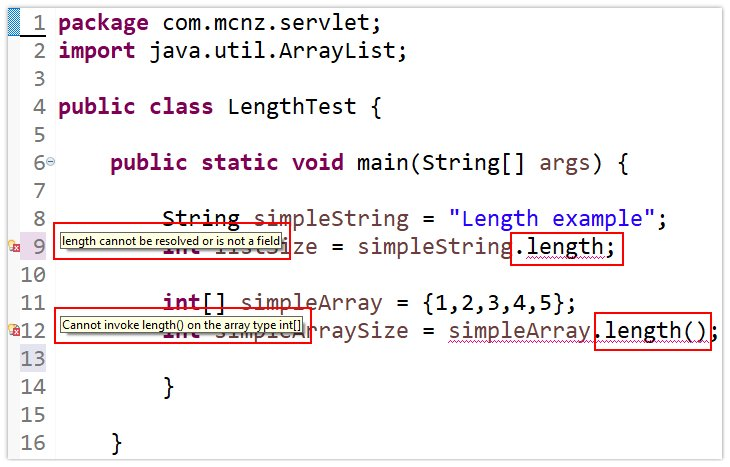# Java array size, length and loop examples

## Java array size and length

Here are the most important concepts developers must know when they size Java arrays and deal with a Java array’s length:

1. The theoretical maximum Java array size is 2,147,483,647 elements.
2. To find the size of a Java array, query an array’s length property.
3. The Java array size is set permanently when the array is initialized.
4. The size or length count of an array in Java includes both null and non-null characters.
5. Unlike the String and ArrayList, Java arrays do not have a size() or length() method, only a length property.

## How do you find the size of an array in Java?

To determine the size of a Java array, query its length property.

Here is an example of how to access the size of a Java array in code:

```int[] exampleArray = {1,2,3,4,5};
int exampleArraySize = exampleArray.length;
System.out.print("This Java array size is: " + exampleArraySize );
//The Java array length example prints out the number 5```

Note that array length in Java is a property, not a method. That means you can’t follow the word length with round brackets.

## How do you size Java arrays?

To set the size of a Java array, explicitly state the intended capacity of the array when you initialize it with the new keyword, as follows:

```// Set the Java array size to three
int[] arraySizeExample = new int;```

Using the new keyword like this permanently sets the Java array size. However, all elements are initially set to null, or to zero if it is an array of primitive types.

Another way to size Java arrays is to provide all of the array elements at the time of initialization:

```// Size the Java array with a set of known values
int[] arraySizeExample = new {0,1,1,2,3,5,8};```

In this case, the size of the Java array is 7. You use the Java array’s length property to print out its size:

```System.out.println("The Java array size is: " + arraySizeExample.length );
```

## What is the maximum Java array size?

When you initialize Java arrays with the new keyword, the size argument is of type int.

The 32-bit Java int can go to a maximum of 2,147,483,647, so that is the theoretical maximum Java array size.

However, virtual machines for different operating systems may not allocate every available bit to the elements in the Java array. Thus, the maximum number of elements a Java array can hold is typically a little less than the upper limit of a Java int.

## Is the Java array size fixed?

In Java, once you set the Java array size it is fixed, and it can’t be changed.

This puts Java in sharp contrast to other programming languages like JavaScript where arrays resize dynamically. But in Java, once the array size is set, it is permanent.

However, there are collection classes in Java that act like a Java array but resize themselves automatically.

Any class that extends the List interface expands dynamically. Java arrays do not expand and contract. You can’t change the size of an array in Java once the array is initialized.

## How is a Java array’s size and length used in a loop?

A common example of the Java array length property being used in code is a program looping through all of the elements in an array.

The following Java array length example prints out every number in an array named fibArray:

```int[] fibArray = {0,1,1,2,3,5,8};
for (int i=0; i < fibArray.length; i++) {
System.out.printlng(fibArray[i]);
}```

## What’s the difference between Java array size vs length?

In common parlance, when people want to know how many elements an array can hold, they use the term ‘size.’

However, Java arrays do not have a size() method, nor do they have a length() method. Instead, the property length provides a Java array’s size.

To further confuse matters, every Java collection class that implements the List interface does have a size() method. Classes including Vector, Stack and ArrayList all have a size method. So to get the size of an ArrayList, you call its size method. However, an Arraylist is not the same thing as a Java array.

To get the size of a Java array, you use the length property. To get the size of an ArrayList, you use the size() method.Know the difference between the Java array length and the String’s length() method.

## What’s the difference between Java array length vs length()?

When you put round brackets at the end of a word in Java, it indicates that you are calling a method.

Without round brackets, you are invoking a method.

The length property tells you the Java array size. It is not a method.

To further confuse matters, the String class does have a length() method. The length method of the String class tells you how many elements a String contains.

Developers often confuse the Java array length property with the Java String’s length() method. The result is a compile time error.You find the Java array size through its length property, not the length() method.

## How do I ask my boss for arrays?

I think you mean ‘a raise.’ That’s more of a professional development issue. But good luck.

I hope that if you do get arrays that it’s a sizeable one, no matter what method you employ, and that your tenure with the company is lengthy.

Close